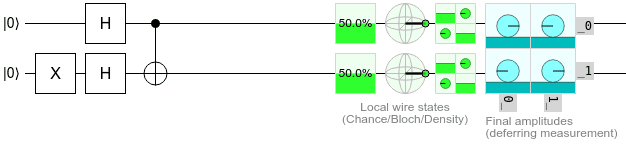# Deutsch–Jozsa algorithm/Circuit Analysis

• A
• bapgobears

#### bapgobears

I'm having a hard time following the analysis of the basic quantum circuit that illustrates Deutsch's Algorithm - essentially I'm not following the algebraic manipulations there making on the two input quantum bits (simple case). One analysis is given at the bottom of the page of the link I provided. I also can not follow the explanation given in section 1.4.3 of the excellent book Quantum Computation and Quantum Information by Michael Nielsen.

I think I understand the basic idea of a qubit and how it can be represented as a complex linear combination of two orthonormal basis states. I can see what a NOT gate does (swaps coefficients) and an H gate (create superpositions), and a CNOT gate although when you start using inputs that are a superposition of basis states I can get confused. For example, the bottom output of the Uf gate in the Duetsch algorithm is suppose to be y XOR f(x).

if y = (|0 - |1)/sqrt(2) and x is (|0 + |1)/sqrt(2) . How do you evaluate

((|0 - |1)/sqrt(2)) XOR f ((|0 + |1)/sqrt(2)) ??

What does it mean to do the XOR of two quantum bits in the general case? If they are computational basis, then that's similar to the classical case I think, but in the case above?

What makes it confusing is sometimes they just write 1 or 0 and not the Dirac notation |1> or |0>. Where they say f(0) - should that really be f(|0>)?

Also, when doing the analysis of multiple input gates they seem to write the states of the individual qubits right next to each other with no punctuation. I can't tell if they mean multiplication sometimes or not?

The funny thing is I more or less get the point they're making that in one measurement you can find out whether the f(x) is balanced or not - something that a classical computer can not do in one calculation, but I can't follow the logic on how they get they're final state.

Any insights, help, or suggestions would be really appreciated. Maybe you know of some good resources that go over more of the fundamentals of quantum circuit analysis?

Thanks,
Brett

https://en.wikipedia.org/wiki/Deutsch–Jozsa_algorithm

It sounds like you haven't quite grasped the implications of the fact that, when you have ##n## 2-level quantum systems (i.e. qubits), the state of the system is a ##2^n##-level quantum system where each level corresponds to one of the ##2^n## values you can assign to ##n## bits.

The expression "((|0 - |1)/sqrt(2)) XOR f ((|0 + |1)/sqrt(2))" makes no sense. The XOR doesn't apply to two qubits and give a single qubit output. It applies inline to each of the components of the superposition, causing a re-arrangement. It works more like...

##\text{CNOT}_{2 \rightarrow 1} \cdot \left( \frac{1}{\sqrt{2}} (|0\rangle - |1\rangle) \otimes \frac{1}{\sqrt{2}} (|0\rangle + |1\rangle) \right)##

Combine the factors of ##\frac{1}{\sqrt{2}}##:

##= \text{CNOT}_{2 \rightarrow 1} \cdot \left( \frac{1}{2} (|0\rangle - |1\rangle) \otimes (|0\rangle + |1\rangle) \right)##

Distribute the tensor product over the subtraction:

##= \text{CNOT}_{2 \rightarrow 1} \cdot \left( \frac{1}{2} \left(|0\rangle\otimes \big(|0\rangle + |1\rangle\big) - |1\rangle\otimes \big(|0\rangle + |1\rangle\big) \right) \right)##

Distribute the tensor products over the additions:

##= \text{CNOT}_{2 \rightarrow 1} \cdot \left( \frac{1}{2} (|0\rangle\otimes |0\rangle + |0\rangle\otimes|1\rangle - |1\rangle\otimes |0\rangle - |1\rangle\otimes|1\rangle) \right)##

Use the equivalent-but-shorter notation ##|ab\rangle## instead of ##|a\rangle \otimes |b\rangle##:

##= \text{CNOT}_{2 \rightarrow 1} \cdot \left( \frac{1}{2} (|00\rangle + |01\rangle - |10\rangle - |11\rangle) \right)##

Distribute the CNOT:

##= \frac{1}{2} (\text{CNOT}_{2 \rightarrow 1} |00\rangle + \text{CNOT}_{2 \rightarrow 1} |01\rangle - \text{CNOT}_{2 \rightarrow 1} |10\rangle - \text{CNOT}_{2 \rightarrow 1} |11\rangle)##

Evaluate the CNOT against each ket (i.e. flip the first bit when the second bit is 1, so 11->01 but 10->10):

##= \frac{1}{2} (|00\rangle + |11\rangle - |10\rangle - |01\rangle)##

Re-order:

##= \frac{1}{2} (|00\rangle - |01\rangle - |10\rangle+ |11\rangle)##

Done.

We can confirm this by simulating in Quirk:The output display shows a positive (rightward) amplitude for 00 and 11, and negative amplitude for 01 and 10. Just like we calculated. (The magnitudes also agree.)

Last edited:
Oh my gosh, thank you Strilanc for putting me out of my misery. You put your finger on precisely my problem! I have not completely grasped the concept of how to work with multiple qubits - how they combine to from a 2^n level system. Your explanation helps a ton. I also found a youtube video just before I got to your post where the steps are explained in more detail, and the notation is a little more complete to help the beginner. I had no idea that a CNOT gate can be thought of as an entangler/detangler. I'm excited and now I can make more progress. That Quirk simulator is cool. Thank you so much! Brett